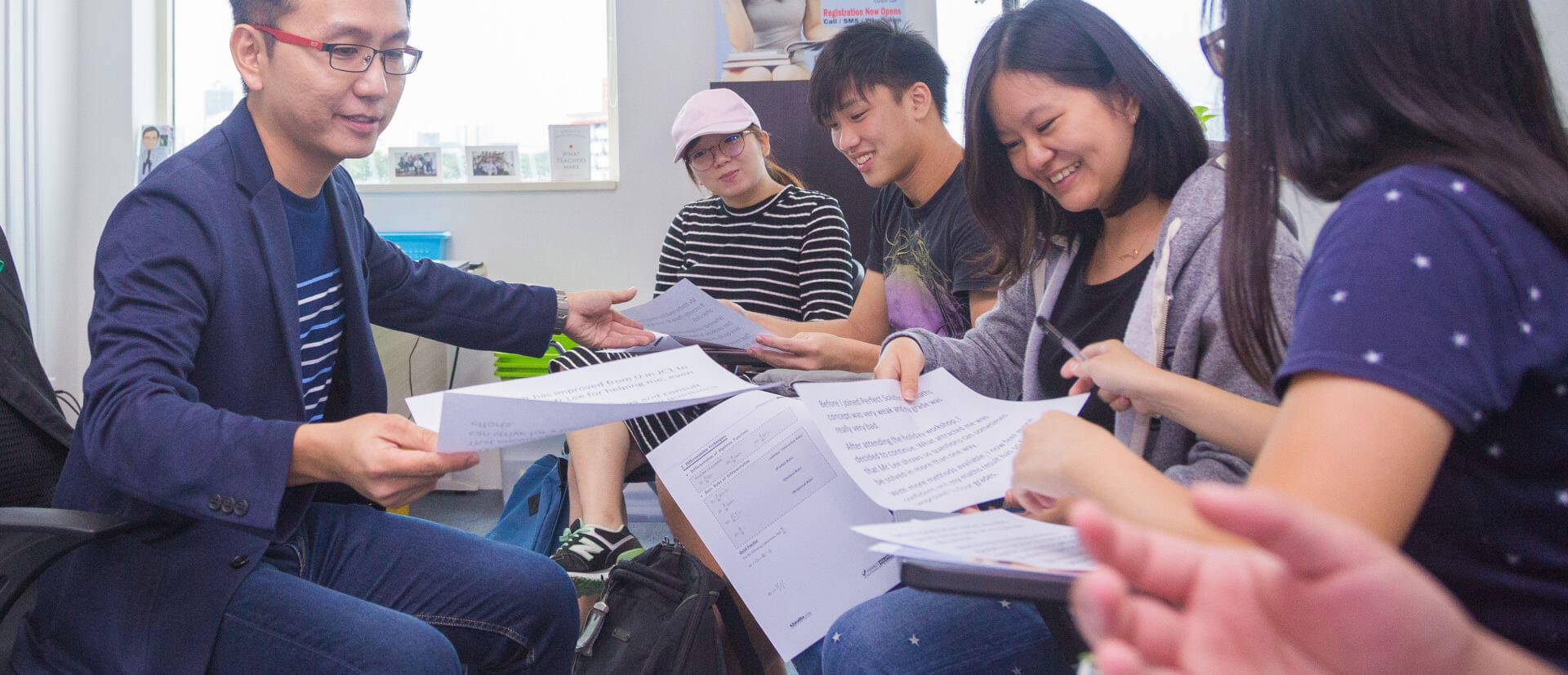# How to choose an Online JC Maths Tutor?

Its mid year and most students are preparing for the inevitable onslaught of tests and exams coming up, especially junior college students. Maths being a subject very dependant on understanding and practice, it helps to have a maths tutor guide and explain difficult concepts.

## Why do I need an online maths tutor?

Due to the recent #circuitbreaker, social distancing was an issue and the only way to have a maths tutor teach us was through the internet. Using softwares like Zoom, Google Hangouts or Skype, an Online JC Maths Tutor could review questions, explain concepts, run through difficult questions, teach new and easier methods on solving questions or simply coach us on how to study effectively.

## How to choose an online maths tutor?

Everyone has different ways of learning. Some require repetition, while others prefer many, many examples and some need visiual explanations like charts and diagrams. When I was in school years ago, I needed a deep understanding of topics so my maths tutor would spend a lot of time with the concepts and background of topics. This helped greatly because I also needed to know “Why”.

### Searching for JC Maths Tutors

The easiest way to search for a tutor is through a internet search engine. A simply search for “JC Maths tutor”, “Online Maths Tutor” or “Online Tutor” will list out the possibilities. Do your research and take note of different online jc maths tutors.

### Shortlisting tutors

Once you have compiled a list of possibilities, the potential tutors have to be shortlisted. The following are my recommendations when shortlisting:

#### Students’ testimonials

One of the best ways to know if a tutor is good is to hear what other students have to say. Be sure to gauge if the testimonials seem authentic and sincere.

#### Students’ performance

Stats is mathematics in its application. Find out how many students has been taught over the years and how well the students fair. At the end of the day, we want to know how much students have gained from a tutor.

Some tutors will provide free study materials, online quizzes and even homework. Yes, homework is boring but getting better at JC maths means practice, practice, practice.

## How do you know if the maths tuition is working?

Grades are the obvious indicators if the maths tuition is working but there are other areas to evaluate. Every maths tutor teaches but what is the added value service or unique selling point. A good maths tutor will share general knowledge, tips and tricks or secrets to solving difficult questions. A good indicator that the maths tuition is working is that you become more knowlegable and have the ability to teach others.

There is no secret to being good at mathematics and maths tuition is not the only approach. One has to regularly practice to get better and consistency is the most important thing.